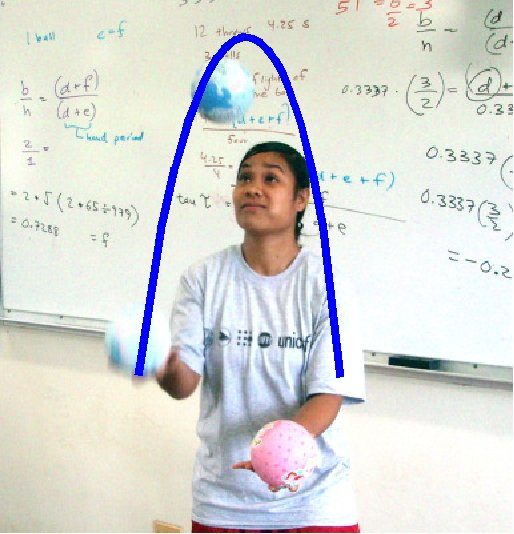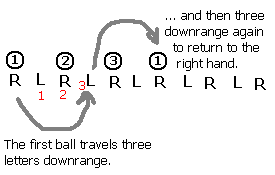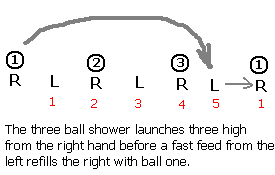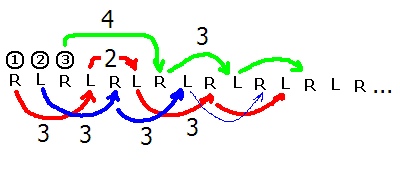# 152 Laboratory 15: Site swap notation

## Question

What is site swap notation?Early in the course a quote from Freeman Dyson was used to start a journey through the mathematical models (relationships) that explain physical systems. Dyson calculated how an electron ought to behave. Later someone went into a laboratory and the electron behaved as predicted by the mathematical model. In laboratory two a linear model predicted the location of a rolling ball. In laboratory three a falling ball obeyed a quadratic mathematical relationship. The behavior of a marble rolling off of a banana leaf obeyed a square root relationship. And in laboratory four the marbles knew what to do in order to mathematically conserve momentum. Sound, the relative depth of an image, and Ohm's law all exhibited linear relationships.

Words for juggling in Micronesia
LanguageJuggle!
Marshalleseejōjō
Kosraenkahkah furoh
Pingalapesemwandimwanda
Mwoakillesemedapwedah
Mortlockese
Northern Noumeneassippo*
Southern Noumeneas
Faichuukese
Satawalese (Yap)
Woleaien
Ulithian
Yapesemachyoch
*Technically a child's game in which a ball is
tossed up and rocks are grabbed in the hand
and then the tossed ball is caught

There are other mathematical relationships that govern physical systems. There are systems that are modeled by exponential, logarithmic, and circular functions. There are exotic functions such as the hyperbolic sine and hyperbolic cosine. Some systems are best described by complex variables that include a real and an imaginary component. Many of these systems are beyond the mathematical scope of this course.

The relationships above are algebraic mathematical models. Much of the mathematics curriculum is centered on algebra in part because algebra is important to describing the physical world. There are, however, other mathematical models, non-algebraic models. This laboratory seeks to broaden your mathematical horizons by introducing a mathematical model and notation that is not algebraic. In this laboratory you will learn about the mathematics of site swaps.

Juggling is the art of keeping more bodies in the air than the number of hands used to loft the bodies. Juggling is an old tradition in Micronesia. Juggling balls follow a parabola in the air. The vertex will be an important concept in this laboratory.

## Site Swap mathematics

Site swap mathematics provides a mathematical notation for juggling that describes the possible juggling patterns for a given number of balls. The numbers are an abstract short hand. The numbers do not tell you how to juggle, the numbers do not tell you where to move your hands, nor whether to throw balls over or under other balls. Site swap notation only tells you to what hand (landing site) the ball will next go.

A simple three ball cascade juggle has each of the three balls passing through each of the hands in alternation. Each hand must touch each ball in succession, with the balls being passed from the right (R) to the left (L) hand and back to the right. The first ball thrown must "fill" the right hand after the third ball has left the right hand. Every ball in this pattern lands three hands (sites) after being thrown.

In the above space-time diagram time is passing as one moves from left to right. R is the right hand, L is the left hand. The hands are the landing "sites," numbered S0, S1, S2… The three balls are indicated by the circles, with the balls numbered from one to three. Note that the first ball thrown lands on the third available landing site S3. S3 is a left hand landing site. Ball one is then launched by the third hand and lands three sites later at S6, a right hand landing site.

The landing site Sn can always be calculated by adding a three to n.

If we "interleave" the left hands inbetween the right hands ...we obtain the following diagram:Studying the above diagram we can see that the number one ball moves three "hands" down the sequence before landing on the left hand (think of hands as landing sites, hence the use of the word site in the name of the notation). The first ball returns to the right hand after moving three more hands (or sites) rightward in the diagram. Remember, the other balls are following the same pattern, landing three sites down.

The pattern above is called a three-ball cascade. In the cascade pattern above each ball lands on the third open site after being thrown. Mathematically this is recorded as 3 3 3 3 3 3 3 3 3... This means each ball lands on the third site down the diagram. To save space, we toss the spaces and write 33333... For further simplicity we invoke a rule that number patterns repeat indefinitely, hence a single 3 can be used to denote this pattern. Thus 3 means 3333333.... forever.

There are four basic patterns in juggling.

• Cascade: each hand throws in alternation to the other hand in a left-right pattern. In the simplest form, balls all rise to the same height.
• Shower: one hand throws the balls in an arc typically higher than a cascade, while the other hands always catches the balls and passes them back to the first hand. The pass back is barely a throw.
• Columns: the objects are juggled in vertical columns. For three balls, this means that one ball moves between the left and right hand while the other two hop up and down staying always on the left or right.
• Fountain: Done with four or more even numbers of balls, the balls always thrown and caught by the same hand.

Another pattern is the three ball shower, with the balls always being thrown from one hand and then caught and fed back to the first hand in a transfer. The right hand must "launch" the balls in sequence "downrange" to the opposite hand. The first opposite open "site" (hand) that permits a transfer (a throw of 1) to set up the relaunch of ball one is the fifth site.Mapping the launches and landings of the other two balls confirms that the balls land "5 site" downrange. This is referred to as a 5 1, or 51 with the spaces removed, with each ball executing the same 51 pattern over and over: 515151...

Other notes:

• Throws alternate hands. The basic site swap system does not take into account synchronous throws.
• A zero is an empty hand.
• Even numbers return to the same hand. A 2 is a ball held in the hand for a "beat", it is not a thrown ball. Think of it as a pause.
• There are n-1 catch/tosses prior to a ball landing.
• Site swap numbers can be conceived of as "beats."
• For the number 3 and above, the height of the throw is related to the number. The relationship is NON-LINEAR.

### The swapping

The notation is called site swap in part because one can "swap" or "switch around" the "sites" (hands!) on which balls land. That it, from a three ball cascade one can toss a ball from the right hand straight up, catching the ball four sites (or four counts or four beats) later. This gives one time to catch and throw a ball from the left hand while the 4 count ball is airborne. While this is happening the left hand holds onto a ball. This is counted as a "2" in the world site swaps (even numbers return to the same hand, holding a ball for an extra beat results in calling this a count of 2).

The diagram depicts a start of a three ball cascade with a single column ball off the right hand followed by a return to a three ball cascade. The notation, unsimplified, would be 33423333...## The math

• For any site swap number sequence the average must be equal to the number of balls.
• Two balls must not come down into the same hand at the same time. This rules out sequences such as a 3 2 1 (321). Any n(n-1) pattern lands two balls in one hand. This rule is far harder to check and generally requires making a space-time diagram or analyzing the orbits of individual balls.

Site swap notation is a mathematical model for juggling, another physical system. Juggling ball orbits are predictable and juggling machines have been successfully build without the need for microprocessors or computers. This single variable mathematical system has rules and systesm of proof. The intent of this laboratory is expand your thinking, to get you to consider any system that you see as potentially having a mathematical model underneath the system.

To determine the number of balls calculate the average for each sequence. Add the numbers as single digits and then divide by the number of numbers. [Note to real site swappers: this is intended as math exercise and the author does not mean to imply that this condition is by any means sufficient. It is not sufficient. The class will not be checking for multiplex landings, transition issues, or excited states.]

Determine the number of balls in the following patterns. If the mean is fractional, then the pattern does not exist or is incomplete.

1. 3 Pattern exists? _______ Number of balls: _________
2. 5 Pattern exists? _______ Number of balls: _________
3. 20 Pattern exists? _______ Number of balls: _________
4. 31 Pattern exists? _______ Number of balls: _________
5. 32 Pattern exists? _______ Number of balls: _________
6. 51 Pattern exists? _______ Number of balls: _________
7. 52 Pattern exists? _______ Number of balls: _________
8. 322 Pattern exists? _______ Number of balls: _________
9. 342 Pattern exists? _______ Number of balls: _________
10. 441 Pattern exists? _______ Number of balls: _________
11. 501 Pattern exists? _______ Number of balls: _________
12. 552 Pattern exists? _______ Number of balls: _________
13. 744 Pattern exists? _______ Number of balls: _________
14. 766 Pattern exists? _______ Number of balls: _________
15. 771 Pattern exists? _______ Number of balls: _________

### Joggling

Running and juggling is difficult with anything other than patterns that synchronize with the cadence of the runner. Asymmetrical patterns such as 51 are very difficult to maintain while running. Since cadence is essentially a two beat, left right proposition, this author suspects that only patterns of single digits N M where N = M are really functional. This is potentially a massive limitation leaving only simple cascades as a functional possibility.

## Equipment

Three balls roughly tennis ball size - bring your own!

## Procedure: Three steps to juggling...

1. First learn to toss one ball back and forth between two hands.
2. Then practice tossing two balls in alternation on a one-two beat from left to right and back again. Do NOT toss both at the same time: wait for the first ball to reach the vertex of its parabola before launching the second ball from the other hand. Practice making both parabolas identical, with a vertex right in front of your forehead.
3. With two balls in your dominant hand, and one in your weak hand, launch ball one from the dominant hand. Wait. Wait. Wait until the ball is at the vertex of the inverted parabola in front of your forehead, then launch the second ball from the left hand under the incoming first ball. Wait for that ball to reach the vertex before launching the third ball under the incoming second ball. The launch is a throw to the inside, causing the vertex of balls thrown by the right hand to peak over the left eye, balls thrown by the left hand should peak over the right eye. Opposite side peak. The most common error is launching balls outside over incoming balls. This is a common difficulty for students who are really good at juggling two mangoes in one hand.